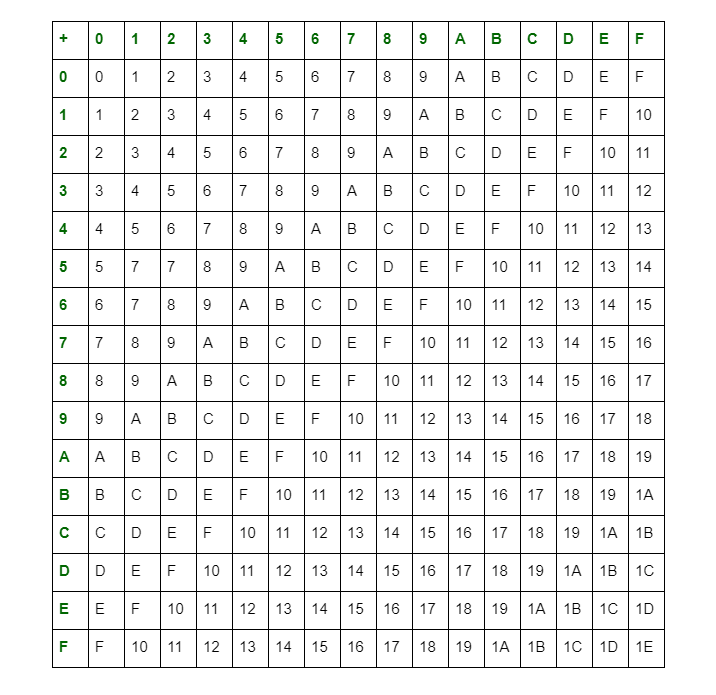# Arithmetic Operations of Hexadecimal Numbers

Hexadecimal Numbers have base of 16 digits ranging from 0 to F (i.e., 0, 1, 2, 3, 4, 5, 6, 7, 8, 9, and A, B, C, D, E, F). A, B, C, D, E, F are equivalent single digits of 10, 11, 12, 13, 14, 15 respectively. Generally, it is expressed by subscript 16 or Hexa (H) or (h).

Arithmetic operations of hexadecimal numbers can be performed using addition table for hexadecimal numbers which is given as below:Arithmetic Operations of Hexadecimal Numbers :

We can perform additions of hexadecimal numbers, with the help of the above table

2. Subtraction :
Subtraction of hexadecimal numbers can be performed by using complement methods or simply as decimal sutractions. The rule of simple hexadecimal subtraction is the digit borrowed from the immediate higher place is counted as 16.

3. Multiplication :
In the multiplication of hexadecimal numbers, if the product is less than radix of hexadecimal (i.e, 16), then we take it as the result, else divide it by radix of hexadecimal (i.e., 16) and take the remainder as the LSB (least significant bit). The quotient is taken as carry in the next significant digit. Using these rules, you can make a table for hexadecimal multiplications.

4. Division :
Similarly, division of hexadecimal numbers can be performed by following the rules of division of decimal numbers but the maximum allowed digit will be F(=15 in decimal).

Arithmetic operations of decimal numbers are very popular and much easier. These operations are also performed as same in other number systems.

Attention reader! Don’t stop learning now. Get hold of all the important DSA concepts with the DSA Self Paced Course at a student-friendly price and become industry ready.

My Personal Notes arrow_drop_upIf you like GeeksforGeeks and would like to contribute, you can also write an article using contribute.geeksforgeeks.org or mail your article to contribute@geeksforgeeks.org. See your article appearing on the GeeksforGeeks main page and help other Geeks.

Please Improve this article if you find anything incorrect by clicking on the "Improve Article" button below.